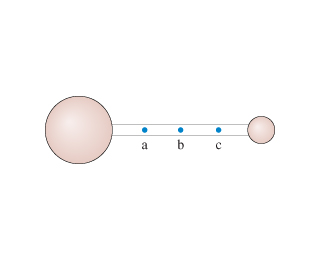# Problem: Is the center of mass of the dumbbell in  at point a, b, or c?

###### FREE Expert Solution

In this problem, we're going to assume that the size of the sphere is proportional to its mass.###### Problem Details

Is the center of mass of the dumbbell in  at point a, b, or c?Frequently Asked Questions

What scientific concept do you need to know in order to solve this problem?

Our tutors have indicated that to solve this problem you will need to apply the Center of Mass & Simple Balance concept. You can view video lessons to learn Center of Mass & Simple Balance. Or if you need more Center of Mass & Simple Balance practice, you can also practice Center of Mass & Simple Balance practice problems.